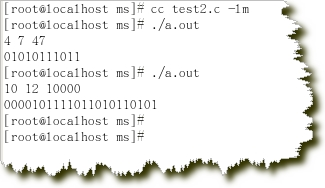# （2014微软实习生笔试题）2.K-th string

Time Limit: 10000ms
Case Time Limit: 1000ms
Memory Limit: 256MB

Description

Consider a string set that each of them consists of {0, 1} only. All strings in the set have the same number of 0s and 1s. Write a program to find and output the K-th string according to the dictionary order. If s​uch a string doesn’t exist, or the input is not valid, please output “Impossible”. For example, if we have two ‘0’s and two ‘1’s, we will have a set with 6 different strings, {0011, 0101, 0110, 1001, 1010, 1100}, and the 4th string is 1001.

Input

The first line of the input file contains a single integer t (1 ≤ t ≤ 10000), the number of test cases, followed by the input data for each test case.
Each test case is 3 integers separated by blank space: N, M(2 <= N + M <= 33 and N , M >= 0), K(1 <= K <= 1000000000). N stands for the number of ‘0’s, M stands for the number of ‘1’s, and K stands for the K-th of string in the set that needs to be printed as output.

Output

For each case, print exactly one line. If the string exists, please print it, otherwise print “Impossible”.

Sample In

3
2 2 2
2 2 7
4 7 47

Sample Out

0101
Impossible
01010111011

=============================================================================================================#include<stdio.h>
#include<stdlib.h>
#include<math.h>
#include<string.h>


//十进制转二进制字符串
char* dec2bin(int n,int k)
{
int i=0;
char* str = (char*)malloc(k*sizeof(char));
for(i=0;i<k;i++)
{
if(1<<i & n)
{
str[k-1-i] = '1';
}
else
{
str[k-1-i] = '0';
}
}
return str;
}


//统计二进制数中1的个数，个数一致则n有效
int is_valid(int n,int s)
{
int c = 0;
for (c = 0; n; ++c)
{
n &= (n -1) ;
}
if(s==c)
{
return 1;
}
else
{
return 0;
}
}

//算法实现函数
void findKth(int n,int m,int K)
{
int tempn = 0;
int count = 0;
char* str;
while(tempn < pow(2,n+m))
{
tempn++;
if(is_valid(tempn,m))
{
count++;
}
if(count==K)
{
str = dec2bin(tempn,n+m);
printf("%s\n",str);
free(str);
return;
}
}
printf("Impossible\n");
}

int main(int argc,char* argv[])
{
int num0=0,num1=0,k=0;
int i=0;
scanf("%d %d %d",&num0,&num1,&k);
findKth(num0,num1,k);

return 0;
}


04-13154506-24650
04-061万+
05-191万+
04-131401
04-171393
04-042328
06-071296
11-156万+
05-178万+
04-121638
10-287120
07-163011
09-1138
04-014140
06-052万+
06-264999
©️2020 CSDN 皮肤主题: 大白 设计师:CSDN官方博客